Function Repository Resource:

# PermutationInvolutionQ

Test whether a permutation equals its inverse

Contributed by: Wolfram Staff
 ResourceFunction["PermutationInvolutionQ"][p] tests whether a permutation p equals its inverse.

## Details

In general, a function f is an involution if f(f(x))=x for all x in the domain of f. For a permutation p, that is equivalent to p=p-1. A permutation is easily seen to be involutive if and only if it is comprised entirely of cycles of lengths 1 or 2.

## Examples

### Basic Examples (2)

A permutation involution with 1↔2, 3↔4:

 In:=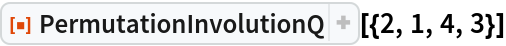Out=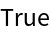The permutation {2,3,1} is not an involution because 1↦2↦31:

 In:=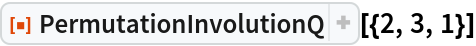Out=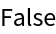### Scope (1)

Test if a permutation in disjoint cyclic format is an involution:

 In:=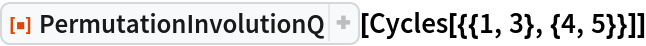Out=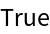### Applications (1)

These are the 10 involutive permutation on 4 elements, shown compactly:

 In:=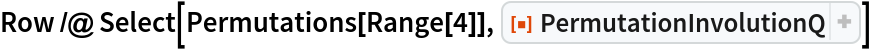Out=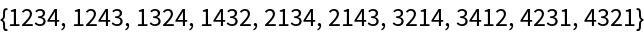George Beck

## Version History

• 1.0.1 – 07 February 2022
• 1.0.0 – 08 July 2019